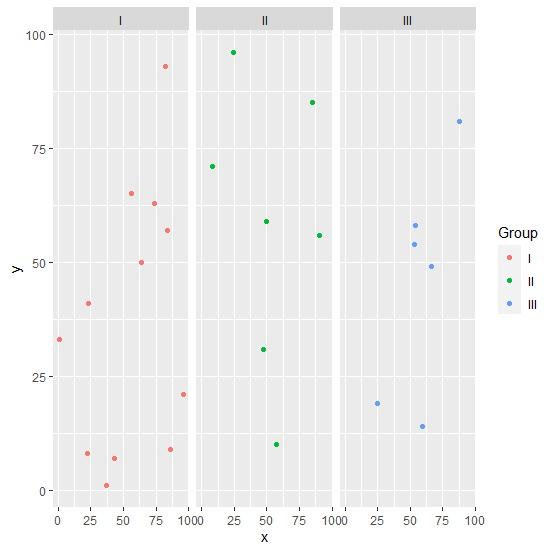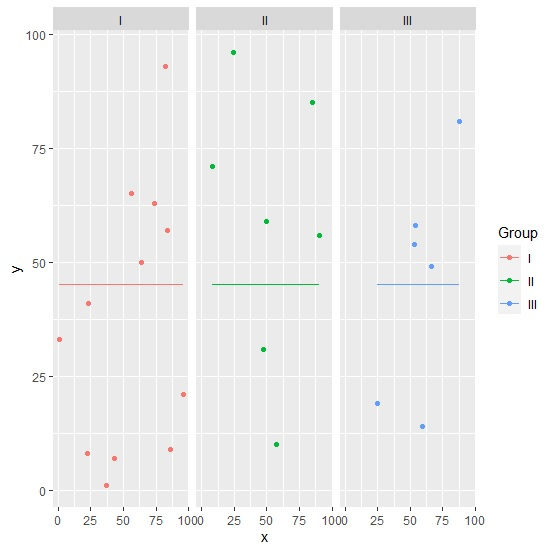# How to display mean line per group in facetted graph using ggplot2 in R?

To display mean per group in facetted graph using ggplot2 in R, we can follow the below steps −

• First of all, create a data frame.

• Then, create the facetted scatterplot between two columns.

• After that, create the facetted scatterplot and add geom_line with mean calculated for y values.

## Create the data frame

Let’s create a data frame as shown below −

Live Demo

x<-sample(1:100,25)
y<-sample(1:100,25)
Group<-sample(c("I","II","III"),25,replace=TRUE)
df<-data.frame(x,y,Group)
df

On executing, the above script generates the below output(this output will vary on your system due to randomization) −

## Output

  x   y  Group
1 24 96   II
2 88 81   III
3 22 8    I
4 50 59   II
5 1 33    I
6 56 65   I
7 57 10   II
8 53 54   III
9 54 58   III
10 66 49  III
11 64 50   I
12 23 41   I
13 84 57   I
14 59 14  III
15 96 21   I
16 86 9    I
17 8 71   II
18 85 85  II
19 47 31  II
20 74 63   I
21 25 19  III
22 90 56  II
23 37 1    I
24 82 93   I
25 43 7    I

## Create facetted scatterplot

Using facete_grid function of ggplot2 package to create the scatterplot between x and y −

x<-sample(1:100,25)
y<-sample(1:100,25)
Group<-sample(c("I","II","III"),25,replace=TRUE)
df<-data.frame(x,y,Group)
library(ggplot2)
ggplot(df,aes(x,y,col=Group))+geom_point()+facet_grid(~Group)

## Output## Display mean line in facetted plot

Using geom_line function and facet_grid function to create the facetted scatterplot between x and y with mean line −

x<-sample(1:100,25)
y<-sample(1:100,25)
Group<-sample(c("I","II","III"),25,replace=TRUE)
df<-data.frame(x,y,Group)
library(ggplot2)
ggplot(df,aes(x,y,col=Group))+geom_point()+facet_grid(~Group)+geom_line(aes(y=mea
n(y)))

## Output